# Analysis of the Screw Flight Wear Model and Wear Regularity of the Bulk Transport in Screw Ship Unloader

## Abstract

The screw flight, spiral blade welded on the axial cylinder, is the core component of the screw ship unloader and can be seriously worn by the materials during long-term conveying. The damaged screw flight will make the screw ship unloader unable to unload materials or even lead to an accident. However, the existing wear model cannot be directly applied to predict the wear of the screw flight under different working conditions. Hence, we established a new screw flight wear model based on the Archard wear model and Continuous Medium Hypothesis to predict the service life of the screw flight. Three influencing factors, including speed, filling rate, and pitch, were selected to study the wear law of the screw flight, and the wear law was verified by EDEM simulation. Results indicate that the simulation results affected by the changes in various factors were consistent with the calculation model. With the increase of rotation speed and filling rate, the screw flight wear rate increased. Nevertheless, with the increase of pitch, the screw flight wear rate first increased and then decreased. The screw flight wear model can be used to calculate the wear rate under different working conditions for the screw flight life prediction.

## Introduction

A vertical screw conveyor is a type of continuous conveying equipment that can lift materials in a short time. The vertical screw conveyor is mainly used in port screw ship unloaders to load and unload coal or crops and has the characteristics of low energy consumption, high transmission efficiency, and capacity to lift more materials (Sun et al. 2018). The vertical screw conveyor is the core component of the screw ship unloader, and its performance directly affects the reliability and service life of the screw ship unloader. The failure of the vertical screw unloader is mainly manifested as the wear of the screw flight. The reason is that the screw flight rotates at a high speed to promote the lifting of the material during operation. Then, the material particles strongly collide with the screw flight, which causes the screw flight to be worn seriously. The severely worn screw flight often leads to downtime accidents, delays production, and reduces the service life of the screw ship unloader.

In recent years, many scholars have conducted researches on the wear of equipment for conveying bulk materials (such as the scraper conveyors and the screw conveyors) and found that the wear types of equipment are mainly abrasive wear but also include fatigue and erosion wear (Moore 1978 ;Piazzetta et al. 2018).

The Archard wear model is a commonly used model to analyze material wear (Archard 1953). Wear coefficient $$K$$, normal contact pressure $$F_{\rm n}$$ and sliding distance $$S$$ are the key factors that constitute the Archard wear model. The wear coefficient $$K$$ is related to the inherent properties of conveying bulk materials, including the {\rm Hardness}, particle shape, and particle size. Many scholars have studied the wear coefficient $$K$$ and sliding distance $$S$$ of different materials based on the Archard wear model. (Bialobrzeska and Kostencki 2015) studied the wear coefficient of low-alloy boron steel based on the Archard wear model and found that during the friction process between particles and low-alloy boron steel, the particles with larger Hardness flake off and cause larger scratches. (Chen et al. 2017) used the Archard wear model to study single-particle sliding wear, and the results indicated that the increase in particle density or radius would cause more steady-state sliding wear at the single-particle level. (Wang et al. 2017) used the Archard wear model to study the wear of the crusher lining by exploring different particle sliding distances, and the numerical calculation showed that the increase in particle sliding distance would increase the crusher wear. (Forsström and Jonsén 2016) combined the DEM-FEM (Discrete Element Method and Finite Element Method) model with the Archard wear model to simulate and analyze the wear position and depth of the dump truck, and the simulation results were consistent with the actual wear of the dump truck which fully proves that the Archard wear model can be used in the research of equipment wear. (Gong et al. 2016) predicted the life of the screw flight in the screw sand washer and used Markov chain Monte Carlo to simulate the random load with the Gauss distribution on the screw flight, and found that the root of the screw flight had stress concentration, and its life decreased exponentially with the increase in residual stress.

The normal contact pressure $$F_{\rm n}$$ is a key factor in the Archard wear model. Normally, the normal contact pressure $$F_{\rm n}$$ is set as a constant in the numerical calculation of material wear. This setting may reduce the accuracy of computing equipment wear. To reduce calculation errors, the EDEM (Discrete Element Method software) which loaded the Archard wear model calculated the force of particle collision on the surface of the equipment at different times and the normal contact pressure $$F_{\rm n}$$ of the equipment at different times by statistics to analyze the wear of the equipment. Wang et al. (2018) used the EDEM software to analysis of the bulk coal transport state of a scraper conveyor and confirmed that the key reason for the failure of the scraper conveyor chutes caused by the coal was abrasive wear. This study proves that the Archard wear model can predict equipment wear well. (Xia et al. 2019) also used EDEM software to study the wear of the scraper conveyor and verified the accuracy of the simulation results through experiments. (Yang 2019) used the EDEM software to study the wear of the screw flight and analyzed the wear of the screw flight under different conditions by changing the filling rate, speed, pitch, and other factors. The results indicated that, as the filling rate increased, the rotation speed became large, the wear of the screw flight increased. The EDEM software based on the discrete element method is used by more scholars to study the wear of equipment for conveying different bulk materials, and the accuracy of its calculation results has been verified by different experiments.

Considering the macroscopic mechanical properties of a large number of particles, the method of obtaining the normal contact pressure of the scattered particles includes the method of Continuous Medium Hypothesis. Janssen (Janssen 1895) proposed to use the continuum model to analyze the static stress of the silo in 1895, and this method has been widely recognized. (Rahmoun and Millet 2008) developed a continuous media approach for the calculation of the stresses in an ensiled granular media which improves on the Janssen theory, and the continuous media approach allowed us to represent qualitatively as well as quantitatively the stress saturation phenomenon in granular silos. (Wang et al. 2015) compared the discrete element comparison with the hydrodynamic approach under the same set of rheological laws, material parameters, numerical method, and the stresses predicted by two approaches match well inflow zones. (Wang et al. 2019) used the Continuous Medium Hypothesis to study the normal contact pressure of the activated coke particles in the absorption tower and verified through experiments that the continuum model can effectively describe the mechanical properties of the activated coke particles in the absorption tower.

Unlike other equipment for conveying bulk materials, the motion state of particles and wear in the screw flight is very complicated. Some scholars (Yang 2019) have studied the wear morphology of screw flight and proposed measures to prevent wear. Nevertheless, there is no wear model in existing studies to predict the wear of screw flight under different operating conditions of the screw ship unloader. Therefore, a well-developed model to predict the service life of the screw flight is very necessary so that the damaged screw flight can be replaced in advance to avoid accidents.

This study established a screw flight wear model based on the Archard wear model which used the Continuous Medium Hypothesis to calculate the normal contact pressure $$F_{\rm n}$$ at different positions of the bulk in the vertical screw conveyor. Then we analyzed the relationship between different influencing factors and screw flight wear. Finally, the model was verified and analyzed through the EDEM simulation.

## Theory and Method

### Model of the Vertical Screw Conveyor

The overall structure of the screw ship unloader is shown in Fig. 1 (Pratap et al. 2017). Its structure mainly includes a vertical screw conveyor, feeding head, horizontal conveyor, truss, and other components (Song et al. 1995). However, the vertical screw conveyor is the core component of the screw ship unloader, and its main structure includes screw flight, shell, motors, and other parts. The process of unloading materials of the screw ship unloader is as follows: first, the screw ship unloader inserts the vertical screw conveyor into the material to be unloaded; second, the material is gathered to the feed port under the push of the feeding head; third, the vertical screw conveyor vertically lifts the material; finally, the horizontal conveyor conveys the material to the scheduled receiving place.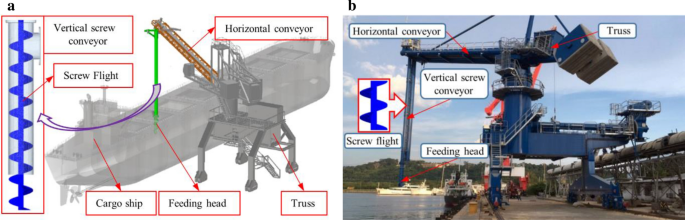Fig. 1

In the process of loading and unloading materials, the strong relative collision between the screw flight and the material causes the screw flight to be worn seriously. For screw ship unloaders, monitoring the wear of screw flight under their working conditions is dangerous and difficult to achieve. Moreover, the screw ship unloaders in different ports have varying working conditions in lifting and conveying different materials, which results in varying wear conditions. Therefore, this study uses simulation methods to predict screw flight wear under various conditions.

### Screw Flight Wear Model

#### The Archard Wear Model

Archard (Archard 1953) established a classic Archard wear model. The Archard wear model mainly expresses a dependence between a removed material and a work done by friction forces. The more the volume of the material removed by the irregular-shaped particles, the more the work done by the friction forces. The Archard wear model is as follows:

$$Q = W \cdot F_{\rm n} \cdot S$$
(1)
$$W = \frac{K}{{\rm Hardness}}$$
(2)

where Q is a volume of material removed, $$K$$ is the dimensionless wear coefficient, $${\rm Hardness}$$ is the surface Hardness of the material, $$F_{\rm n}$$ is the normal contact pressure at the wear location, and $$S$$ is a sliding distance.

#### Establishment of the Screw Flight Wear Model

The wear model of the screw flight can be established, which is based on the Archard wear model and the Continuous Medium Hypothesis of the coal particles in the vertical screw conveyor. The sliding distance of the particles per unit time is calculated by the single-particle method, and the normal contact pressure $${F}_{\rm n}$$ is calculated by the Continuous Medium Hypothesis.

1. (1)

First, we should calculate the sliding distance of the particles per unit time. When the material begins to exhibit the limit of vertical upward movement, the force acting on the particle where the surface of the screw flight contacts the shell is shown in Fig. 2 under the rotation of the screw flight.

As shown in Fig. 2, the screw tangent plane (nt) and the screw normal plane (nb) along the screw plane direction are set at the point P where the particle is located. The t is the tangential direction in the screw tangent plane (nt), the b is the normal direction in the normal plane (nb) of the screw, and the n is the normal direction of point P relative to the screw shaft. The $${f}_{\rm n}$$ is the supporting force of the screw conveyor shell to the particle, the $${f}_{\rm b}$$ is the supporting force of the screw flight to the particle, and the $${f}_{\rm t}$$ is the resultant force of the friction generated by the particle, the screw flight surface, and the screw conveyor shell.

In this extreme case, gravity, centrifugal force, and frictional forces on the particles should be in equilibrium.

$$m \cdot {\text{g}} \cdot \left( {\sin \alpha + \mu_{p} \cdot \cos \alpha } \right) = \frac{{m \cdot v_{k}^{2} }}{r} \cdot \mu_{g} \cdot \left( {\cos \alpha - \mu_{p} \cdot \sin \alpha } \right)$$
(3)

According to the Moiré stress circle diagram of particles in bulk mechanics (Knuth et al. 2012), the maximum ratio of shear stress $$\tau$$ to compressive stress $$\sigma$$ is the internal friction coefficient $$\mu_{\rm p}$$ of the material.

$$\mu_{p} = {\text{tan}}\beta = \left( {\frac{\tau }{\sigma }} \right)_{{{\text{max}}}}$$
(4)

According to Eqs. (3 and 4), the circumferential velocity $$v_{\rm k}$$ of the particle under this limit is.

$$v_{k} = \sqrt {\frac{r \cdot g}{{\mu_{g} }}{\text{tan}}\left( {\alpha + \beta } \right)} ,$$
(5)

where $$m$$ is the mass of the particle, $$r$$ is the screw flight radius,$${ }\mu_{\rm p}$$ is the coefficient of friction between the particle and the screw flight, $$\mu_{\rm g}$$ is the coefficient of friction between the particle and the screw conveyor shell, $$\alpha$$ is the inclination angle of the spiral surface in Fig. 2, and $${ }\beta$$ is the friction angle between the particle and the spiral surface.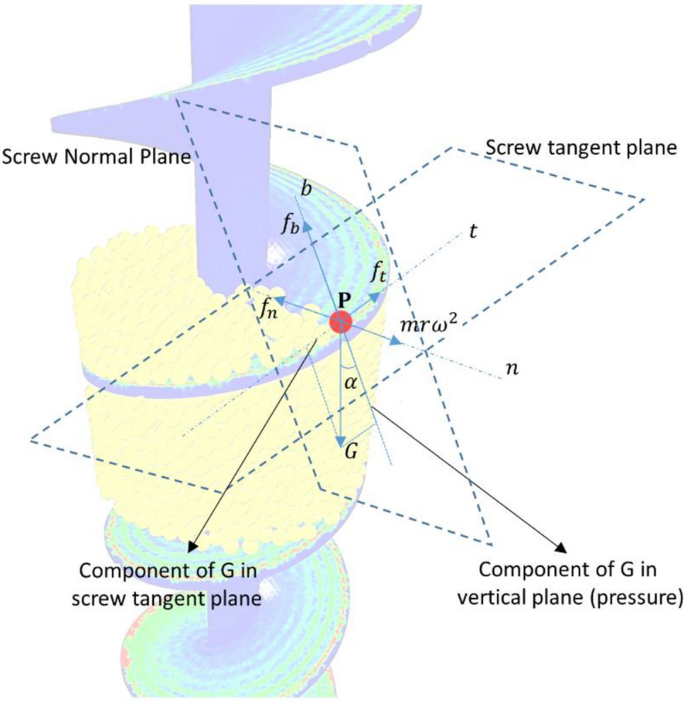Fig. 2

The speed of the particle is vs. Thus, the relative speed vsk between the particle phase and the screw flight is

$$v_{\text{sk}} = (v_s - v_k)\cdot \text{cos}\;\alpha.$$
(6)

The relative displacement of particle in a unit of time is

$$\text{d}s=v_{\text{sk}}\cdot \text{d}t,$$
(7)
$$\text{d}s=\left(v_s-\sqrt {\frac{r \cdot g}{{\mu_{g} }}{\text{tan}}\left( {\alpha + \beta } \right)}\right)\text{cos}\;\alpha\cdot \text{d}t.$$
(8)
2. (2)

Secondly, the Continuous Medium Hypothesis is used to analyze the normal contact pressure $$F_{\rm n}$$ at different positions of the equipment. As shown in Fig. 3a, the normal contact pressure on the screw flight at the point Q is the normal contact pressure of all particles in the $${\text{d}} \rm r$$ width above the Q point, as follows:

$$F_{\rm n} = M \cdot g \cdot \cos \alpha + \frac{{M \cdot v_{k}^{2} }}{r} \cdot \mu_{s} \cdot \sin \alpha ,$$
(9)
$$M = \rho \cdot g \cdot k\left( r \right) \cdot \frac{\pi }{4} \cdot D_{p}^{2} ,$$
(10)

where $$M$$ is the total mass of all particles stacked above a certain point of the screw flight, $$k\left( r \right)$$ is the particle stack height in Fig. 3a, the $$\rho$$ is the particle density, and the $$\mu_{\rm s}$$ is the sliding friction coefficient between the particles.

The actual screw conveying material cannot fill the entire vertical screw conveyor and moves to the side of the shell under the action of centrifugal force. The filling types of the material under the same screw parameters are shown in Fig. 3a–d.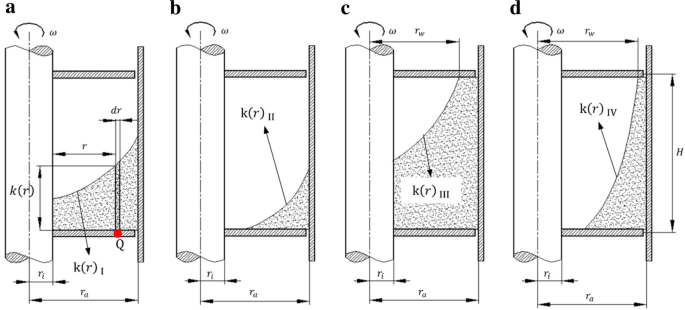Fig. 3

The $$k\left( r \right)$$ is solved following the parabolic equation of the rotating liquid proposed by Gabler (1981) as the initial equation theory. Equation is as follows:

$$k\left( r \right) = k^{*} + \frac{{\omega^{2} }}{2g} \cdot r^{2} ,$$
(11)

where $$k^{*}$$ is determined by the method of calculating the volume of the rotating body in the Guldinschen Regel law (Neuendorff 1919). Equation is as follows:

$$V = 2 \cdot \pi \cdot \mathop \smallint \limits_{{r_{i} }}^{{r_{a} }} r \cdot k\left( r \right) \cdot {\text{d}}r,$$
(12)
$$V = \varphi \cdot \left( {r_{a}^{2} - r_{i}^{2} } \right) \cdot \pi H,$$
(13)
$$V = 2 \cdot \pi \cdot \mathop \smallint \limits_{{r_{i} }}^{{r_{a} }} r \cdot \left( {k^{*} + \frac{{\omega^{2} }}{2g} \cdot r^{2} } \right) \cdot {\text{d}}r = \varphi \cdot \left( {r_{a}^{2} - r_{i}^{2} } \right) \cdot \pi \cdot H.$$
(14)

Using Eqs. (810), the wear model of the screw flight can be established as

$${\text{d}}Q = W \cdot F_{\rm n} \cdot {\text{d}}S$$
(15)
\begin{aligned} \frac{{{\text{d}}Q}}{{{\text{d}}t}} & = \frac{K}{{\rm Hardness}} \cdot \rho \cdot g \cdot k\left( r \right) \cdot \frac{\pi }{4} \cdot D_{p}^{2} \left( {g \cdot \cos \alpha + \frac{{v_{k}^{2} }}{r} \cdot \mu_{s} \cdot \sin \alpha } \right) \\ & \quad .\left( {v_{s} - \sqrt {\frac{r \cdot g}{{\mu_{g} }}{\text{tan}}\left( {\alpha + \beta } \right)} } \right)\cos \alpha . \\ \end{aligned}
(16)

According to the different material filling types in Fig. 3, $$k\left(r\right)$$ can be calculated by Eqs. (1114) as

$$\left\{ \begin{gathered} {\text{k}}\left( r \right)_{{\text{ I}}} = \varphi \cdot H - \frac{{\omega^{2} }}{4 \cdot g} \cdot \left( {r_{a}^{2} + r_{i}^{2} } \right) + \frac{{\omega^{2} }}{2 \cdot g} \cdot r^{2} \hfill \\ {\text{k}}\left( r \right)_{{{\text{II}}}} = \omega \cdot r_{a} \cdot \sqrt {\frac{{H \cdot \varphi \cdot \left( {r_{a}^{2} - r_{i}^{2} } \right)}}{{g \cdot r_{a}^{2} }}} - \frac{{\omega^{2} }}{2 \cdot g} \cdot \left( {r_{a}^{2} - r^{2} } \right) \hfill \\ {\text{k}}\left( r \right)_{{\text{ III}}} = H - \omega \cdot \sqrt {\frac{H}{g} \cdot \left[ {r_{a}^{2} \cdot \left( {1 - \varphi \cdot \frac{{r_{a}^{2} - r_{i}^{2} }}{{r_{a}^{2} }}} \right) - r_{i}^{2} } \right]} + \frac{{\omega^{2} }}{2 \cdot g} \cdot \left( {r^{2} - r_{w}^{2} } \right) \hfill \\ {\text{k}}\left( r \right)_{{\text{ IV}}} = H + \frac{{\omega^{2} }}{2 \cdot g} \cdot \left[ {r^{2} - \frac{H \cdot g}{{\omega^{2} }} - r_{a}^{2} \cdot \left( {1 - \varphi \cdot \frac{{r_{a}^{2} - r_{i}^{2} }}{{r_{a}^{2} }}} \right)} \right], \hfill \\ \end{gathered} \right.$$
(17)

where $$\varphi$$ is the filling rate of the material in the screw flight, $$H$$ is the pitch, $$\omega$$ is the rotation speed of the screw flight, $$r_{i}$$ is the radius of the screw shaft, and $$r_{a}$$ is the radius of the screw flight.

Among them, as shown in Table 1, the type of k(r) can be judged by the material filling rate in the vertical screw conveyor.

### Theoretical Analysis and Calculation of Screw Flight Wear Model

In the established theoretical model of screw flight wear, the wear rate of the screw flight is related to the wear coefficient K, the pitch of the screw flight, the speed of the screw flight, the material filling rate, the particle diameter, the density, and the running time of the vertical screw conveyor.

This paper is mainly based on the screw flight wear model to study the screw flight wear law under different working conditions. In the analysis of the influence of physical parameters on the wear performance of the screw conveyor, two key variables, the screw flight speed $$\omega$$, and the material filling rate $$\varphi$$, are used to study the influence of this model on the wear rate. In the analysis of the influence of geometric factors on the wear of the screw conveyor, the influence of the wear rate of the model is analyzed by selecting the change in the pitch $$H$$ of the screw flight.

This paper selects coal to study the wear of a screw flight. The material of screw flight is Q235A plain carbon structural steel. Because the surface temperature of the screw flight does not change much during the coal conveying process, its Hardness can be regarded as a constant. The friction factor between coal and screw flight is selected within the applicable range. Hence, the wear coefficient K in the Archard wear model can be set to a constant value of 3.685e-4, and not as the main factor in study.

In this paper, the LX-200 screw ship unloader is selected for the wear research. The screw ship unloader is rated to transport 1500 tons of coal per hour. The diameter of the screw flight is 200 mm and the length is 3000 mm. The parameters of the screw flight are as follows in Table 2. The diameter of the coal particles is selected to be 16 mm, the density $$\rho$$ is $$1500{\text{kg}}/{\text{m}}^{3}$$, the friction angle $$\beta$$ between the particles and the screw flight is 21.77°, and the sliding friction coefficient between the particles $$\mu_{\rm s}$$ is 0.6.

Therefore, according to the initial conditions shown in Table 3, the effects of the rotation speed $$\omega$$, the material filling rate $$\varphi$$, and the pitch $$H$$ on the wear rate in the screw flight wear model are analyzed.

## EDEM Simulation Verification

### Simulation Model of Vertical Screw Conveyor

The vertical screw conveyor model is established using 3D software of the SolidWorks. Then, the model is imported into EDEM, as shown in Fig. 4. The material and structure parameters of its vertical screw conveyor are shown in Table 4 (Sun et al. (Sun et al. 2017)).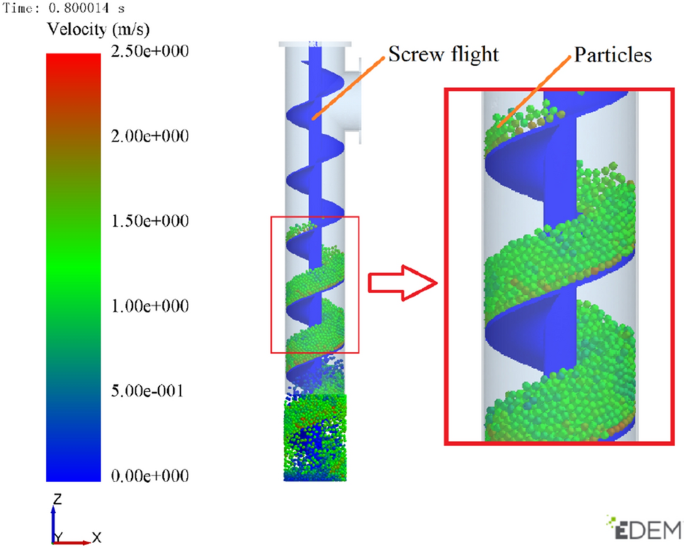Fig. 4

### Determination of the Particle Contact Model

In this study, the Hertz–Mindlin (No Slip) model is used as the contact model between particles (Mindlin (Mindlin 1953)). For the softball contact model, the Hertz–Mindlin contact model is a model commonly used to describe the collision between two particles. It is a nonlinear contact model, as shown in Fig. 5, which is based on normal and tangential contact stiffness, damping, and Coulomb friction. Carious rolling friction models can also be described (Zhao et al. (Zhao et al. 2018)). Table 5 presents the contact characteristic parameters (Wang et al. (Wang et al. 2018)).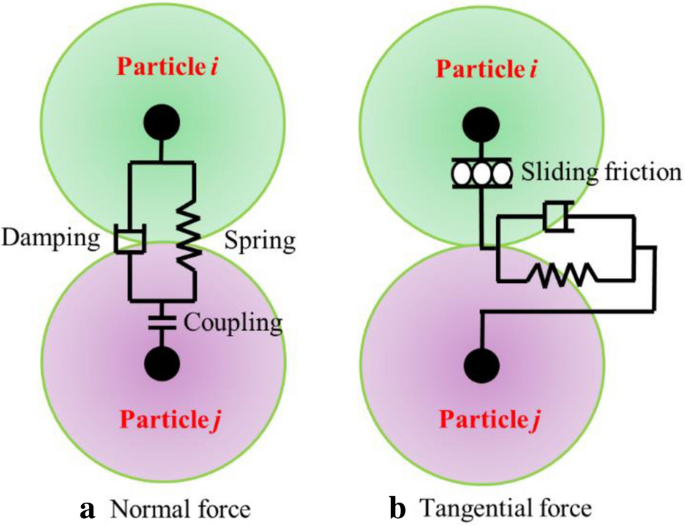Fig. 5

With the assumption that particles $$i$$ and $$j$$ with radius $$R_{1}$$ and $$R_{2}$$ collide, respectively, the normal force $$F_{{{\text{np}}}}$$ and tangential force $$F_{{{\text{tp}}}}$$ between the particles are as follows:

$$F_{{{\text{np}}}} = \frac{4}{3} \cdot E^{*} \cdot \left( {R^{*} } \right)^{\frac{1}{2}} \cdot \theta^{\frac{3}{2}} ,$$
(18)
$$F_{{{\text{tp}}}} = - S_{{{\text{tp}}}} \cdot \delta_{{{\text{tp}}}} ,$$
(19)

where $$E^{*}$$ is the equivalent elastic modulus, $$R^{*}$$ is the equivalent particle radius, $$\theta$$ is the normal overlap, $$S_{{{\text{tp}}}}$$ is the tangential stiffness, and $$\delta_{{{\text{tp}}}}$$ is the tangential displacement.

The normal damping force $$F_{{{\text{np}}}}^{{\text{d}}}$$ and tangential damping $$F_{{{\text{tp}}}}^{{\text{d}}}$$ are as follows:

$$F_{{\rm np}}^{{\rm d}} = - 2\sqrt{\frac{5}{6}} \cdot \beta \cdot \sqrt {S_{np} \cdot m^{*} } \cdot v_{{\rm np}}^{{\rm rel}} ,$$
(20)
$$F_{{\rm tp}}^{{\rm d}} = - 2\sqrt{\frac{5}{6}} \cdot \beta \cdot \sqrt {S_{{\rm tp}} \cdot m^{*} } \cdot v_{{\rm tp}}^{{\rm rel}} ,$$
(21)
$${\upbeta } = \frac{\ln \varepsilon }{{\left( {\ln \varepsilon } \right)^{2} + \pi^{2} }},$$
(22)

where $$m^{*}$$ is the equivalent mass, $$S_{{{\text{np}}}}$$ is the normal stiffness, $$v_{{{\text{np}}}}^{{{\text{rel}}}}$$ is the normal relative velocity, $$v_{{{\text{tp}}}}^{{{\text{rel}}}}$$ is the tangential relative velocity, and $$\varepsilon$$ is the recovery coefficient.

### Determination of the Simulation Parameters Under Different Working Conditions

In the EDEM simulation, the parameters of rotation speed, filling rate, and pitch are adjusted separately for simulation, and the 12 sets of simulation parameters are shown in Table 6 below. The simulation time is set to 10 s. Then, the area within one pitch of the screw flight middle section is intercepted to observe the wear of the screw flight.

### EDEM Simulation Results

#### Screw Flight Wear Under Different Rotation Speeds

The observation in Fig. 6 indicates that the average wear depth of the screw flight of the vertical screw conveyor under different rotation speeds increases with the increase of the speed. The most severe wear occurs at the outer edge of the screw flight, and the amount of wear on the inner side of the screw shaft is small.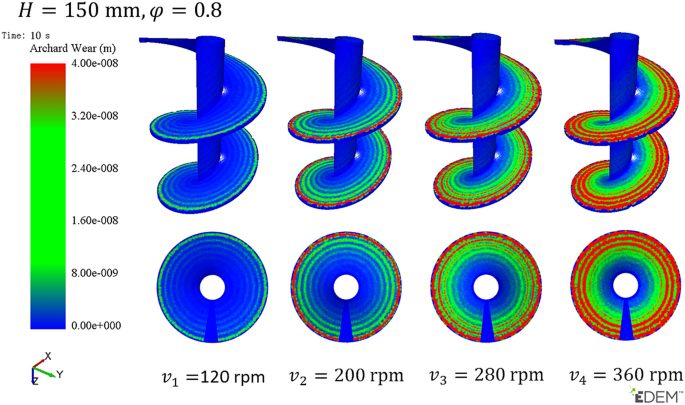Fig. 6

The average and maximum wear of screw flight at four different rotation speeds of 120, 200, 280, and 360 rpm are shown in Fig. 7. This Figure shows that the average depth of wear of the screw flight increases as time prolongs. The average and maximum wear amounts of the screw flight are the maximum when the rotation speed is 360 rpm. When the screw flight rotation speed is 360 rpm, the average wear depth is 1.47e-08 m and the maximum wear value is 5.04e-07 m at 10 s. The average wear of screw flight at 200, 280, and 360 rpm increases by 68%, 210%, and 449% relative to the average wear of screw flight at 120 rpm. The higher rotation speed leads to greater wear of the screw flight, and the growth trend of wear becomes higher as the rotation speed increases. The increase of rotation speed leads to the increase of mass flow of material at the same time. And the increase of relative rotational speed between coal and screw flight accelerates the surface wear of screw flight. There are small fluctuations of the curves in Fig. 7b. The discrete element simulation software EDEM counts the collision force between all particles, which records a set of maximum wear result every 0.1 s, the particle collision force at the maximum wear position is different at different times, the fluctuant normal contact pressure leads to fluctuations in the maximum wear depth.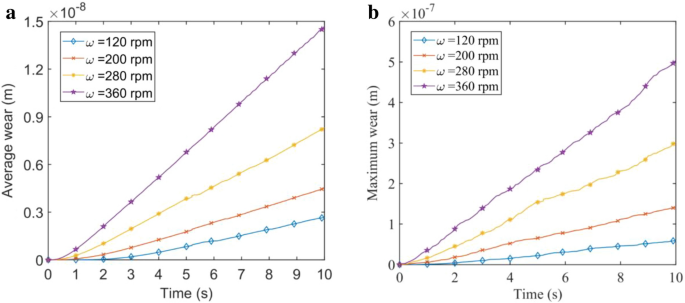Fig. 7

#### Screw Flight Wear Under Different Filling Rates

Figure 8 shows the changing trend of the wear depth of the screw flight under different filling rates. As the filling rate increases, the wear position of the screw flight gradually spreads from the outside to the inside. Moreover, when the screw flight rotation speed is 280 rpm with different filling rates, the amount of wear on the outermost side of the screw flight only slightly changes.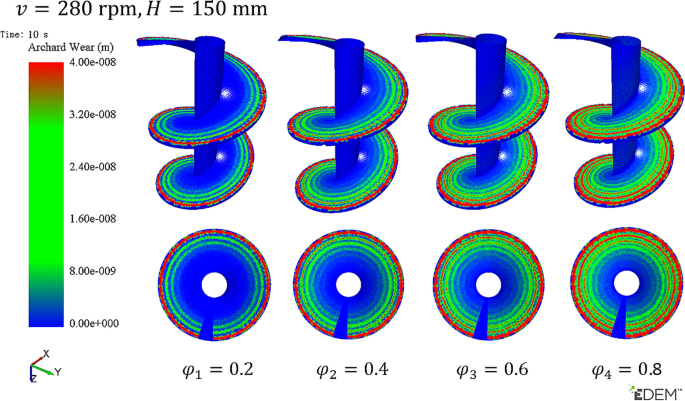Fig. 8

The average and maximum wear of screw flight at four different filling rates of 0.2, 0.4, 0.6, and 0.8 are shown in Fig. 9. This Figure indicates that the average and maximum wear increase linearly as time prolongs. At the filling rate of 0.8, the average and maximum wear of the screw flight are the maximum. When the filling rate is 0.8, the average wear depth is 8.71e-09 m and the maximum wear value is 3.09e-07 m at 10 s. The average wear at the filling rates of 0.4, 0.6, and 0.8 is 1.53, 2.19, and 3.21 times that at the filling rate of 0.2. As the filling rate increases, the wear of the screw flight also increases, but the growth trend of the wear differs. A large filling rate corresponds to a fast rate of increase in wear. On the contrary, a small filling rate indicates a slow rate of increase in wear. Results proves that with the increase of the filling rate, the normal contact pressure $${F}_{n}$$ acting on the surface of the screw flight increases, resulting in the intensified wear of the screw flight.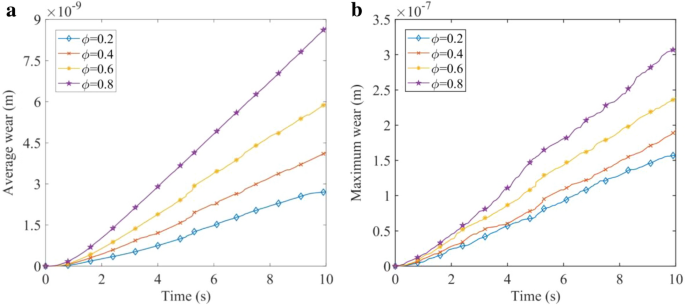Fig. 9

#### Screw Flight Wear Under Different Pitches

Figure 10 shows the screw flight wear under different pitch factors. With the increase in the pitch, the wear of the screw flight increases, and the direction of screw flight wear consistent with the rotation direction of the screw flight. When the pitches are 200 and 225 mm, the screw shaft on the screw flight wears under the spiral direction.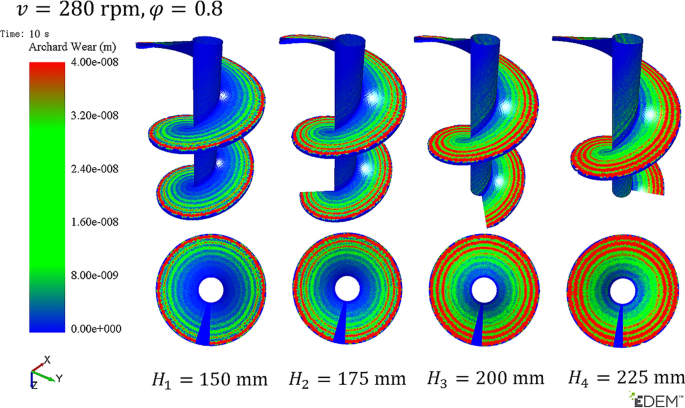Fig. 10

Figure 11 shows the average and maximum wear of screw flights with pitches of 150, 175, 200, and 225 mm. Regardless of the pitch, the average and maximum wear of the screw flight continue to increase as time prolongs. With the change in the pitch, the difference in the average wear of the screw flight is smaller than that of the rotation speed and the pitch.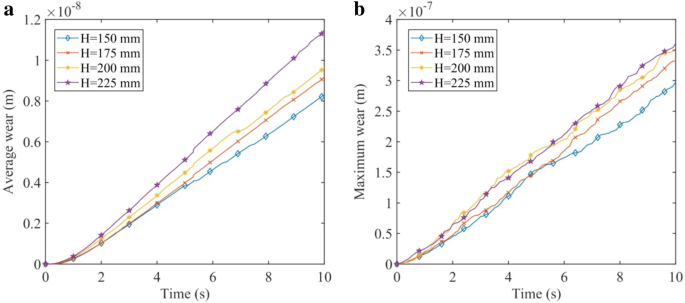Fig. 11

The curves of average and maximum wear in the first 5 s of different pitches intersect, which indicates that the wear of the screw flight caused by the pitch factor in the first 5 s is not the main factor. After 5 s, the amount of wear caused by different pitch factors gradually differentiates with the increase in time.

Therefore, with the increase in the pitch, the wear of the screw flight gradually increases, but its wear rate is low. The pitch factor also influences the wear of the screw flight but is not the main factor.

## Contrast Analysis of Screw Flight Theoretical Wear Model and Simulation Results

According to the result of the EDEM simulation, the average wear of the screw flight within one pitch under different working conditions can be obtained.

Figure 12 marks four groups of line segments (Line 1- Line 4) along the normal direction of the spiral axis and passing through the screw flight surface. Observe the EDEM simulation results, the point wear on the screw flight under the same radius with the spiral shaft as the center is almost the same. Therefore, it can be assumed that the average wear of the screw flight on Plan1 is the same as the average wear within one pitch.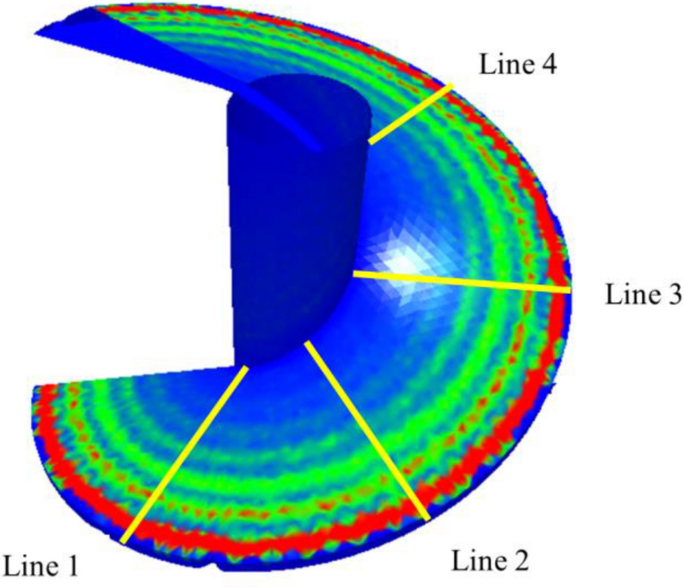Fig. 12

According to the established screw flight wear model, the average wear rate of the radial line (Line 1), from outer edge to screw shaft along the normal direction n, is derived:

\begin{aligned} \frac{{{\text{d}}Q}}{{{\text{d}}t}}_{average} & = \frac{K}{{\rm Hardness}}\left( {g \cdot \cos \alpha + \frac{{v_{k}^{2} }}{r} \cdot \mu_{s} \cdot \sin \alpha } \right) \cdot \left( {v_{s} - \sqrt {\frac{r \cdot g}{{\mu_{g} }}{\text{tan}}\left( {\alpha + \beta } \right)} } \right)\cos \alpha \cdot \rho \cdot g \\ & \quad \cdot \frac{{\mathop \smallint \nolimits_{{r_{i} }}^{{r_{a} }} k\left( r \right){\text{d}}r}}{{\left( {r_{a} - r_{i} } \right)}} \cdot \frac{\pi }{4} \cdot D_{p}^{2} \\ \end{aligned}
(23)

The average wear rate of the screw flight on Line 1 can be calculated by MATLAB. And the following results can be obtained. Figure 13 shows the relationship between the rotation speed $$\omega$$, filling rate $$\varphi$$, pitch $$H$$, and wear rate in the screw flight wear model. Figure 13a presents the relationship between the rotation speed and the wear rate of the screw flight. With the increase in the rotation speed, the trend of the wear rate increases from slow to fast. When the filling rate $$\varphi$$ is 0.8 and the pitch $$H$$ is 200 mm, the wear rate increases the fastest. The wear rate reaches the maximum value of 1.953e-9 when the rotation speed $$\omega$$ is 400 rpm. The increase of the rotational speed, which results in the increase of the displacement that the particle wear spiral surface under the same running time, leads to more wear. Figure 13b shows the relationship between the filling and screw flight wear rates. As the filling rate increases, the wear rate increases linearly. When the rotation speed $$\omega$$ is 280 rpm and the pitch $$H$$ is 200 mm, the slope of the curve is the largest and the growth rate is the fastest. The screw flight wear rate reaches a maximum of 1.347e-9 when the filling rate $$\varphi$$ is 1. With the increase of the filling rate, which increases positive pressure $$F_{\rm n}$$ acting on the surface of screw flight, the wear rate of screw flight increases linearly in theoretical model. Figure 13c shows the relationship between the pitch and the wear rate of the screw flight. As the pitch increases, the wear rate of the screw flight increases first and then decreases. Under different initial conditions, the increase and decrease in the screw flight wear rate differ, and the pitch $$H$$ corresponding to the maximum wear rate also differs. When the rotation speed $$\omega$$ is 280 rpm and the filling rate $$\varphi$$ is 0.8, the growth rate of this curve is the largest. The screw flight wear rate reaches the maximum 1.201e-9 when the pitch $$H$$ is 288 mm. The wear rate of screw flight was higher when the pitch was 200 mm-350 mm. As the pitch increased, the inclination angle α of the spiral surface increased (Fig. 2). Based on Eq. (9), the normal contact pressure $$F_{\rm n}$$ at the wear location increases first and then decreases, leading to the wear rate first increase and then decrease.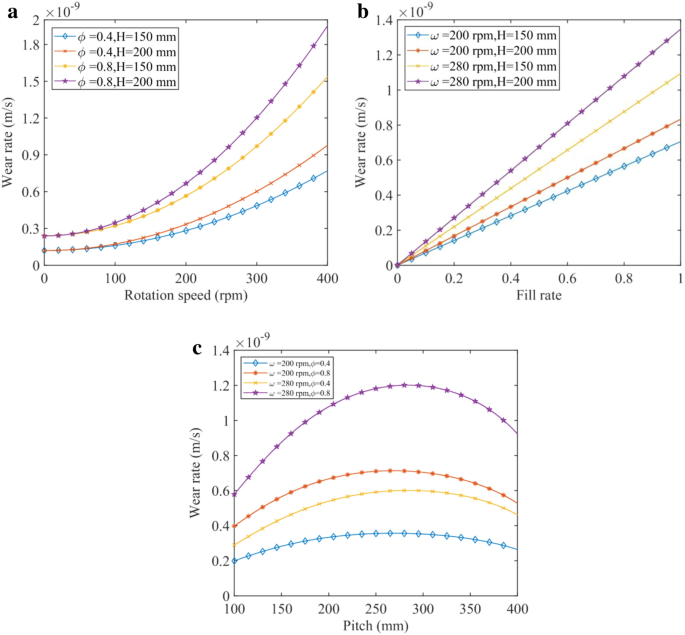Fig. 13

As shown in Fig. 14, according to the operating parameters of the screw ship unloader under different working conditions, the theoretical calculation results are compared with the EDEM simulation results. The theoretically calculated wear curve, the simulated wear curve, and the fitting curve of the simulation data are compared in Fig. 14a–c and the error percentage between the theoretical and simulation is shown in Fig. 14d–f with the change in the rotation speed, filling rate, and pitch. The comparison shows that the obtained simulation data are very close to the theoretical data. It is found that the error percentage between theoretical calculation and simulation is finally maintained at ± 20%. The error is within the acceptable range. Although some graphs show that the theoretically calculated wear curve intersects the fitted curve of the simulation data, the difference between the slopes of the two straight lines is very small. This finding indicates that the wear rate data are very close. The screw flight theoretical model can provide theoretical support for the screw flight wear analysis of the vertical screw conveyor.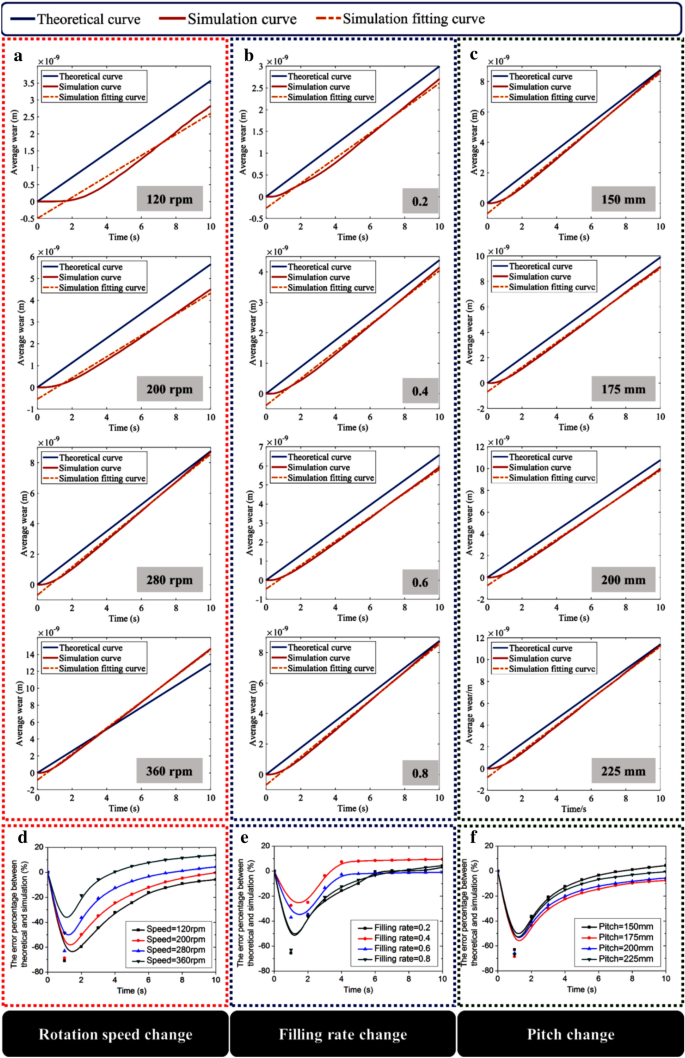Fig. 14

The data comparison between the theoretical and simulated wear rates of the screw flight under different rotation speeds, filling rates, and pitches are shown in Table 7. The maximum error percentage indicates that the theoretical wear rate calculated by the screw flight wear model is in good agreement with the simulation results. Therefore, the screw flight wear model can effectively predict the wear of the screw flight, quickly estimate the amount of product wear, and support the design of the vertical screw conveyor.

## Conclusion

Based on the Archard wear model and the Continuous Medium Hypothesis of the coal particles in the vertical screw conveyor, this paper has constructed the screw flight wear model and simulated it in EDEM by controlling the influence variables. The following conclusions are obtained:

1. (1)

The proposed screw flight wear model was established based on the Archard wear theory which obtaining the normal contact pressure of the scattered particles based on the method of Continuous Medium Hypothesis:

$$\frac{{{\text{d}}Q}}{{{\text{d}}t}}_{average} = \frac{K}{{\rm Hardness}}\left( {g \cdot \cos \alpha + \frac{{v_{k}^{2} }}{r} \cdot \mu_{s} \cdot \sin \alpha } \right) \cdot \left( {v_{s} - \sqrt {\frac{r \cdot g}{{\mu_{g} }}{\text{tan}}\left( {\alpha + \beta } \right)} } \right)\cos \alpha \cdot \rho \cdot g \cdot \frac{{\mathop \smallint \nolimits_{{r_{i} }}^{{r_{a} }} k\left( r \right){\text{d}}r}}{{\left( {r_{a} - r_{i} } \right)}} \cdot \frac{\pi }{4} \cdot D_{p}^{2} .$$ The model analyzed the correlation between the screw flight wear rate and the screw flight speed, material filling rate, pitch, particle diameter, particle density, and other factors.

1. (2)

In the screw flight wear model, two operating parameters of the screw ship unloader, rotation speed and filling rate, were selected to verify the accuracy of the relationship between the operating parameters and the wear rate. Results showed that the wear rate of screw flight was approximately parabola increased with the increase of rotational speed and the screw flight wear rate positively and linearly correlated with the filling rate.

2. (3)

The influence of the pitch in the screw flight wear model, the design parameter in the production of the screw ship unloader, was studied and verified by simulation. Results showed that the screw flight wear rate first increased and then decreased with the increased pitch. The wear rate of screw flight was higher when the pitch was 200 mm-350 mm.

## Data Availability

The data used to support the findings of this study are available from the corresponding author upon request.

## Abbreviations

Q :

Volume of material removed

$$K$$ :

Wear coefficient

$${\rm Hardness}$$ :

Hardness of the screw flight material

$$F_{\rm n}$$ :

Normal contact pressure

$$S$$ :

Sliding distance

$$\mu_{\rm p}$$ :

Friction Coefficient between particle and screw flight

$$\mu_{\rm g}$$ :

Friction Coefficient between particle and conveyor shell

$$\mu_{\rm s}$$ :

Sliding friction coefficient between the particles

$$\alpha$$ :

Inclination angle of spiral surface

$$\beta$$ :

Friction angle between particle and the spiral surface

$$r_{\rm i}$$ :

Radius of the screw shaft

$$r_{\rm a}$$ :

Radius of the screw flight

$$\tau$$ :

Shear stress

$$\sigma$$ :

Compressive stress

$$\rho$$ :

Particle density

$$\omega$$ :

Rotation speed of screw flight

$$\varphi$$ :

Filling rate

$$H$$ :

Pitch

$$v_{\rm s}$$ :

Speed of particle

$$v_{\rm k}$$ :

Speed of screw flight

$$v_{\rm sk}$$ :

Relative speed between particle phase and screw flight

$$F_{{{\text{np}}}}$$ :

Normal force of particle

$$F_{{{\rm {tp}}}}$$ :

Tangential force of particle

$$E^{*}$$ :

Equivalent elastic modulus

$$R^{*}$$ :

Equivalent particle radius

$$S_{{{\rm {tp}}}}$$ :

Tangential stiffness

$$\delta_{{{\rm {tp}}}}$$ :

Tangential displacement

$$\varepsilon$$ :

Recovery coefficient

## References

1. Archard JF (1953) Contact and rubbing of flat surfaces. J Appl Phys 24(8):981–988

2. Bialobrzeska B, Kostencki P (2015) Abrasive wear characteristics of selected low-alloy boron steels as measured in both field experiments and laboratory tests. Wear 328:149–159

3. Chen G, Schott DL, Lodewijks G (2017) Sensitivity analysis of DEM prediction for sliding wear by single iron ore particle. Eng Comput 34(6):2031–2053

4. Forsström D, Jonsén P (2016) Calibration and validation of a large scale abrasive wear model by coupling DEM-FEM: local failure prediction from abrasive wear of tipper bodies during unloading of granular material. Eng Fail Anal 66:274–283

5. Gabler H (1981) Theoretische und experimentelle untersuchungen der forderung in steilen und senkrechten schneckenforderern. Dissertation TU Munich 1981:12–50

6. Gong J, Fu YF, Xia W, Li JH, Zhan F (2016) Fatigue life prediction of screw blade in screw sand washing machine under random load with gauss distribution. Am J Eng Appl Sci 9(4):1198–1212

7. Günthner WA (2003) Empirische entwicklung von kenngrößen zur auslegung von hochleistungs-schneckenförderern für schüttgut. AT Aufbereitungs-Technik 1(10):32–61

8. Janssen HA (1895) Versuche Über Getreidedruck in Silozellen. Verein Deutscher Ingenieur 39(35):1045–1049

9. Knuth MA, Johnson JB, Hopkins MA, Sullivan RJ, Moore JM (2012) Discrete element modeling of a mars exploration rover wheel in granular material. J Terrramech 49(1):27–36

10. Mindlin RD (1953) Elastic spheres in contact under varying oblique forces. J Applied Mech 20:327–344

11. Moore MA (1978) Abrasive wear. Int J Mater Eng Appl 1(2):97–111

12. Neuendorff R (1919) Die guldinschen regeln. Lehrbuch der Mathematik. Springer, Berlin, pp 200–204

13. Piazzetta GR, Lagoeiro LE, Figueira IFR, Rabelo MAG, Pintaude G (2018) Identification of abrasion regimes based on mechanisms of wear on the steel stylus used in the Cerchar abrasiveness test. Wear 410:181–189

14. Pratap S, Nayak A, Kumar A, Cheikhrouhou N, Tiwari MK (2017) An integrated decision support system for berth and ship unloader allocation in bulk material handling port. Comput Ind Eng 106:386–399

15. Rahmoun J, Millet O, De Saxcé G (2008) A continuous media approach to modeling the stress saturation effect in granular silos. J Stat Mech Theory Exp 2008(06):1–19

16. Song JZ, Feng G, Yuefeng H (1995) Lifting behavior of multi-thread screw ship unloader. J Dali Univ Technol 35(2):192–197

17. Sun XX, Meng WJ, Yuan Y (2017) Design method of a vertical screw conveyor based on Taylor–Couette–Poiseuille stable helical vortex. Adv Mech Eng 9(7):1–11

18. Sun XX, Meng WJ, Yuan Y (2018) Conditions of gas–solid two-phase flow formed in a vertical screw conveyor. Adv Mech Eng 10(9):1–10

19. Wang Y, Lu Y, Ooi JY (2015) A numerical study of wall pressure and granular flow in a flat-bottomed silo. Powder Technol 282:43–54

20. Wang Z, Wang R, Fei Q, Li J, Tang J, Shi B (2017) Structure and microscopic wear analysis of lining material based on EDEM. MATEC Web Conf 128:03001–03004

21. Wang X, Li B, Yang Z (2018) Analysis of the bulk coal transport state of a scraper conveyor using the discrete element method. Stroj Vestn J Mech Eng 64(1):37–46

22. Wang RZ, Mao FZ, Fang X, Huang Z, Wang B (2019) Analysis of equivalent continuum model about activated coke particle in absorption tower. J Heilongjiang Inst Technol Chin 19(12):84–89

23. Xia R, Wang X, Li B, Wei X, Yang Z (2019) Discrete element method-(DEM-) based study on the wear mechanism and wear regularity in scraper conveyor chutes. Math Probl Eng 2019:1–12

24. Yang JH (2019) Study on abrasion of screw conveyor screw in sand mixing equipment. Petroleum Chem Equip Chin 4:5–9

25. Zhao S, Evans TM, Zhou X (2018) Effects of curvature-related DEM contact model on the macro-and micro-mechanical behaviours of granular soils. Géotechnique 68(12):1085–1098

Download references

## Acknowledgements

The authors disclosed receipt of the following financial support for the research, authorship, and/or publication of this article: This study was also financially supported by the National Natural Science Foundation Project of China under Grant 52075356, 2019 Key R & D project (International Scientific and Technological Cooperation) of Shanxi Province under Grant 201903D421005, Natural Science foundation of Shanxi Province under Grant 201901D111246, Natural Science Foundation of Shanxi Province under Grant 201901D111236, and Graduate Education Innovation Project of Shanxi Province under Grant 2019BY119.

## Author information

Authors

### Corresponding author

Correspondence to Wenjun Meng.

## Ethics declarations

### Conflicts of interest

The authors declare that they have no conflicts of interest.

## Rights and permissions

Open Access This article is licensed under a Creative Commons Attribution 4.0 International License, which permits use, sharing, adaptation, distribution and reproduction in any medium or format, as long as you give appropriate credit to the original author(s) and the source, provide a link to the Creative Commons licence, and indicate if changes were made. The images or other third party material in this article are included in the article's Creative Commons licence, unless indicated otherwise in a credit line to the material. If material is not included in the article's Creative Commons licence and your intended use is not permitted by statutory regulation or exceeds the permitted use, you will need to obtain permission directly from the copyright holder. To view a copy of this licence, visit http://creativecommons.org/licenses/by/4.0/.

Reprints and Permissions